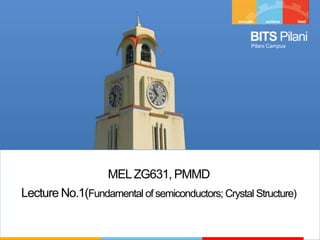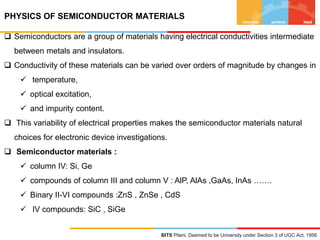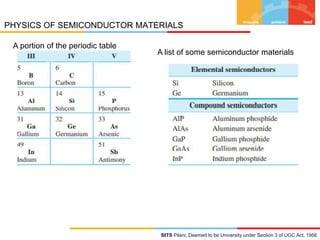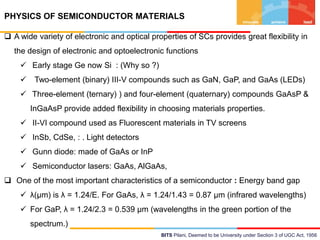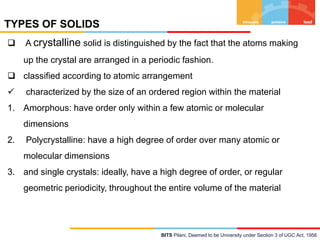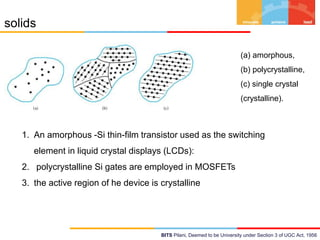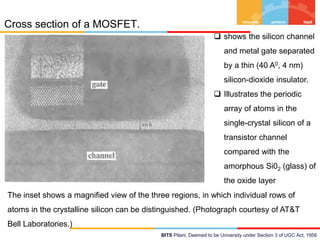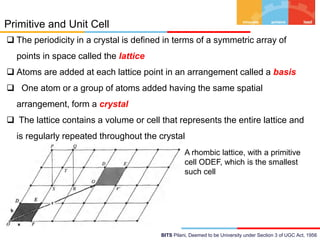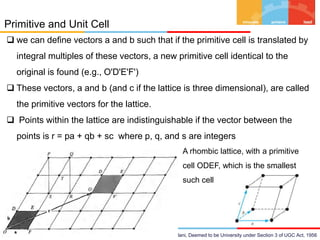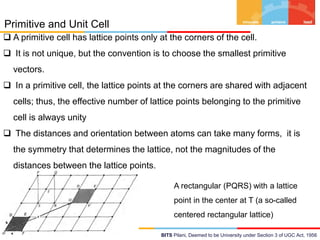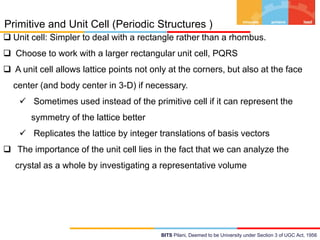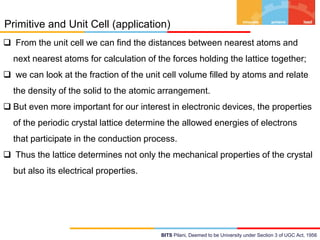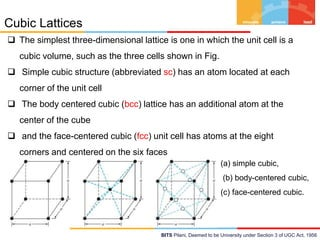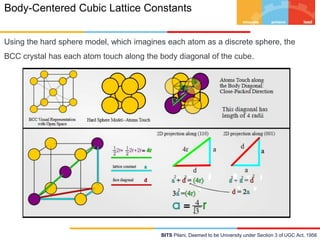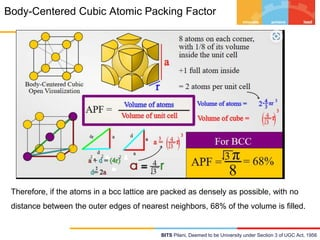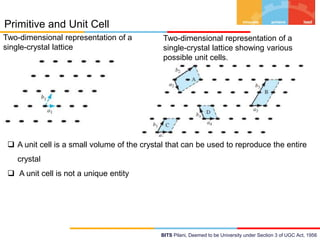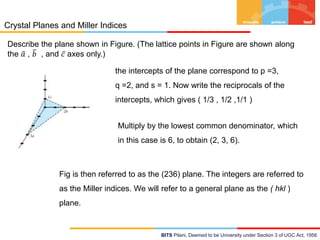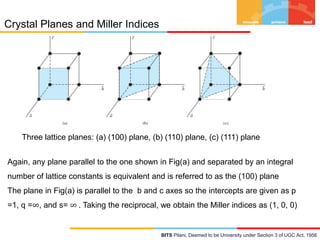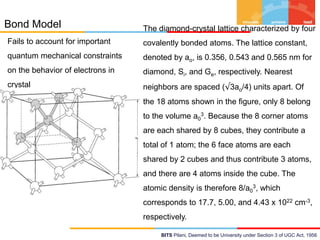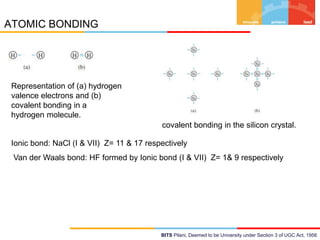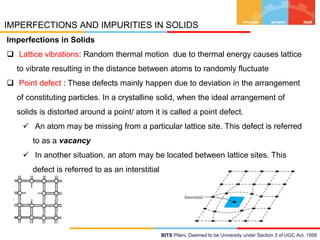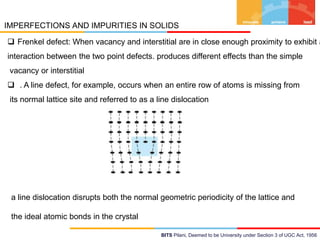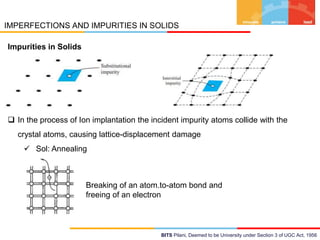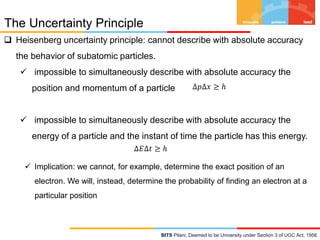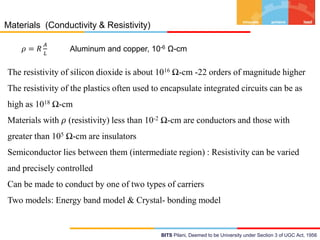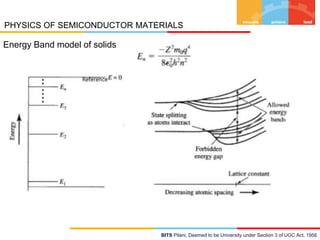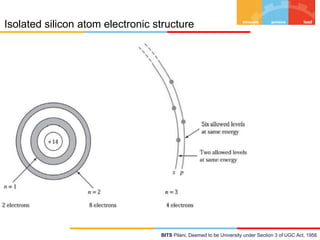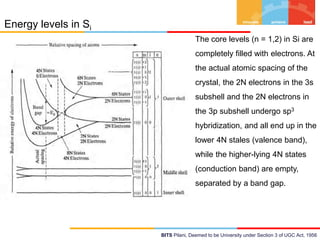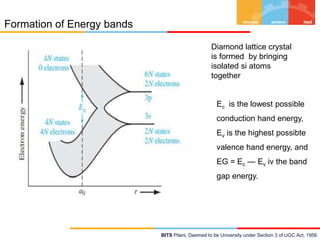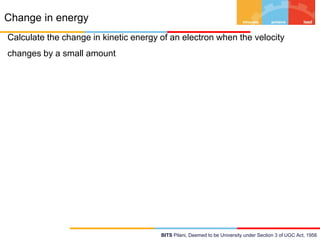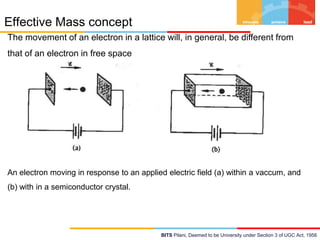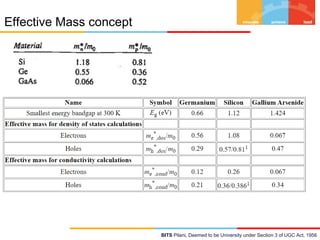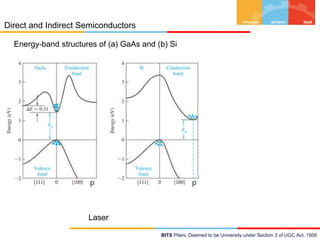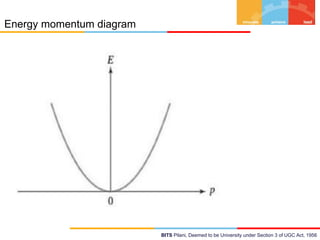1 de 35

### Lecture1_PMMD_effectivemass.pptx

1. BITS Pilani Pilani Campus BITS Pilani presentation R.K.Tiwary EEE Rk.Tiwari@pilani.bits-pilani.ac.in
2. BITS Pilani Pilani Campus MELZG631, PMMD Lecture No.1(Fundamental of semiconductors; Crystal Structure)
3. BITS Pilani, Deemed to be University under Section 3 of UGC Act, 1956 PHYSICS OF SEMICONDUCTOR MATERIALS  Semiconductors are a group of materials having electrical conductivities intermediate between metals and insulators.  Conductivity of these materials can be varied over orders of magnitude by changes in  temperature,  optical excitation,  and impurity content.  This variability of electrical properties makes the semiconductor materials natural choices for electronic device investigations.  Semiconductor materials :  column IV: Si, Ge  compounds of column III and column V : AlP, AlAs ,GaAs, InAs …….  Binary II-VI compounds :ZnS , ZnSe , CdS  IV compounds: SiC , SiGe
4. BITS Pilani, Deemed to be University under Section 3 of UGC Act, 1956 PHYSICS OF SEMICONDUCTOR MATERIALS A portion of the periodic table A list of some semiconductor materials
5. BITS Pilani, Deemed to be University under Section 3 of UGC Act, 1956 PHYSICS OF SEMICONDUCTOR MATERIALS  A wide variety of electronic and optical properties of SCs provides great flexibility in the design of electronic and optoelectronic functions  Early stage Ge now Si : (Why so ?)  Two-element (binary) III-V compounds such as GaN, GaP, and GaAs (LEDs)  Three-element (ternary) ) and four-element (quaternary) compounds GaAsP & InGaAsP provide added flexibility in choosing materials properties.  II-VI compound used as Fluorescent materials in TV screens  InSb, CdSe, : . Light detectors  Gunn diode: made of GaAs or InP  Semiconductor lasers: GaAs, AlGaAs,  One of the most important characteristics of a semiconductor : Energy band gap  λ(µm) is λ = 1.24/E. For GaAs, λ = 1.24/1.43 = 0.87 µm (infrared wavelengths)  For GaP, λ = 1.24/2.3 = 0.539 µm (wavelengths in the green portion of the spectrum.)
6. BITS Pilani, Deemed to be University under Section 3 of UGC Act, 1956 TYPES OF SOLIDS  A crystalline solid is distinguished by the fact that the atoms making up the crystal are arranged in a periodic fashion.  classified according to atomic arrangement  characterized by the size of an ordered region within the material 1. Amorphous: have order only within a few atomic or molecular dimensions 2. Polycrystalline: have a high degree of order over many atomic or molecular dimensions 3. and single crystals: ideally, have a high degree of order, or regular geometric periodicity, throughout the entire volume of the material
7. BITS Pilani, Deemed to be University under Section 3 of UGC Act, 1956 solids (a) amorphous, (b) polycrystalline, (c) single crystal (crystalline). 1. An amorphous -Si thin-film transistor used as the switching element in liquid crystal displays (LCDs): 2. polycrystalline Si gates are employed in MOSFETs 3. the active region of he device is crystalline
8. BITS Pilani, Deemed to be University under Section 3 of UGC Act, 1956 Cross section of a MOSFET. The inset shows a magnified view of the three regions, in which individual rows of atoms in the crystalline silicon can be distinguished. (Photograph courtesy of AT&T Bell Laboratories.)  shows the silicon channel and metal gate separated by a thin (40 A0, 4 nm) silicon-dioxide insulator.  Illustrates the periodic array of atoms in the single-crystal silicon of a transistor channel compared with the amorphous Si02 (glass) of the oxide layer
9. BITS Pilani, Deemed to be University under Section 3 of UGC Act, 1956 Primitive and Unit Cell  The periodicity in a crystal is defined in terms of a symmetric array of points in space called the lattice  Atoms are added at each lattice point in an arrangement called a basis  One atom or a group of atoms added having the same spatial arrangement, form a crystal  The lattice contains a volume or cell that represents the entire lattice and is regularly repeated throughout the crystal A rhombic lattice, with a primitive cell ODEF, which is the smallest such cell
10. BITS Pilani, Deemed to be University under Section 3 of UGC Act, 1956 Primitive and Unit Cell  we can define vectors a and b such that if the primitive cell is translated by integral multiples of these vectors, a new primitive cell identical to the original is found (e.g., O'D'E'F')  These vectors, a and b (and c if the lattice is three dimensional), are called the primitive vectors for the lattice.  Points within the lattice are indistinguishable if the vector between the points is r = pa + qb + sc where p, q, and s are integers A rhombic lattice, with a primitive cell ODEF, which is the smallest such cell
11. BITS Pilani, Deemed to be University under Section 3 of UGC Act, 1956 Primitive and Unit Cell A rectangular (PQRS) with a lattice point in the center at T (a so-called centered rectangular lattice)  A primitive cell has lattice points only at the corners of the cell.  It is not unique, but the convention is to choose the smallest primitive vectors.  In a primitive cell, the lattice points at the corners are shared with adjacent cells; thus, the effective number of lattice points belonging to the primitive cell is always unity  The distances and orientation between atoms can take many forms, it is the symmetry that determines the lattice, not the magnitudes of the distances between the lattice points.
12. BITS Pilani, Deemed to be University under Section 3 of UGC Act, 1956 Primitive and Unit Cell (Periodic Structures )  Unit cell: Simpler to deal with a rectangle rather than a rhombus.  Choose to work with a larger rectangular unit cell, PQRS  A unit cell allows lattice points not only at the corners, but also at the face center (and body center in 3-D) if necessary.  Sometimes used instead of the primitive cell if it can represent the symmetry of the lattice better  Replicates the lattice by integer translations of basis vectors  The importance of the unit cell lies in the fact that we can analyze the crystal as a whole by investigating a representative volume
13. BITS Pilani, Deemed to be University under Section 3 of UGC Act, 1956 Primitive and Unit Cell (application)  From the unit cell we can find the distances between nearest atoms and next nearest atoms for calculation of the forces holding the lattice together;  we can look at the fraction of the unit cell volume filled by atoms and relate the density of the solid to the atomic arrangement.  But even more important for our interest in electronic devices, the properties of the periodic crystal lattice determine the allowed energies of electrons that participate in the conduction process.  Thus the lattice determines not only the mechanical properties of the crystal but also its electrical properties.
14. BITS Pilani, Deemed to be University under Section 3 of UGC Act, 1956 Cubic Lattices  The simplest three-dimensional lattice is one in which the unit cell is a cubic volume, such as the three cells shown in Fig.  Simple cubic structure (abbreviated sc) has an atom located at each corner of the unit cell  The body centered cubic (bcc) lattice has an additional atom at the center of the cube  and the face-centered cubic (fcc) unit cell has atoms at the eight corners and centered on the six faces (a) simple cubic, (b) body-centered cubic, (c) face-centered cubic.
15. BITS Pilani, Deemed to be University under Section 3 of UGC Act, 1956 Body-Centered Cubic Lattice Constants Using the hard sphere model, which imagines each atom as a discrete sphere, the BCC crystal has each atom touch along the body diagonal of the cube.
16. BITS Pilani, Deemed to be University under Section 3 of UGC Act, 1956 Body-Centered Cubic Atomic Packing Factor Therefore, if the atoms in a bcc lattice are packed as densely as possible, with no distance between the outer edges of nearest neighbors, 68% of the volume is filled.
17. BITS Pilani, Deemed to be University under Section 3 of UGC Act, 1956 Primitive and Unit Cell Two-dimensional representation of a single-crystal lattice Two-dimensional representation of a single-crystal lattice showing various possible unit cells.  A unit cell is a small volume of the crystal that can be used to reproduce the entire crystal  A unit cell is not a unique entity
18. BITS Pilani, Deemed to be University under Section 3 of UGC Act, 1956 Crystal Planes and Miller Indices Describe the plane shown in Figure. (The lattice points in Figure are shown along the 𝑎 , 𝑏 , and 𝑐 axes only.) the intercepts of the plane correspond to p =3, q =2, and s = 1. Now write the reciprocals of the intercepts, which gives ( 1/3 , 1/2 ,1/1 ) Multiply by the lowest common denominator, which in this case is 6, to obtain (2, 3, 6). Fig is then referred to as the (236) plane. The integers are referred to as the Miller indices. We will refer to a general plane as the ( hkl ) plane.
19. BITS Pilani, Deemed to be University under Section 3 of UGC Act, 1956 Crystal Planes and Miller Indices Three lattice planes: (a) (100) plane, (b) (110) plane, (c) (111) plane Again, any plane parallel to the one shown in Fig(a) and separated by an integral number of lattice constants is equivalent and is referred to as the (100) plane The plane in Fig(a) is parallel to the b and c axes so the intercepts are given as p =1, q =∞, and s= ∞ . Taking the reciprocal, we obtain the Miller indices as (1, 0, 0)
20. BITS Pilani, Deemed to be University under Section 3 of UGC Act, 1956 Bond Model Fails to account for important quantum mechanical constraints on the behavior of electrons in crystal The diamond-crystal lattice characterized by four covalently bonded atoms. The lattice constant, denoted by ao, is 0.356, 0.543 and 0.565 nm for diamond, Si, and Ge, respectively. Nearest neighbors are spaced (√3ao/4) units apart. Of the 18 atoms shown in the figure, only 8 belong to the volume a0 3. Because the 8 corner atoms are each shared by 8 cubes, they contribute a total of 1 atom; the 6 face atoms are each shared by 2 cubes and thus contribute 3 atoms, and there are 4 atoms inside the cube. The atomic density is therefore 8/a0 3, which corresponds to 17.7, 5.00, and 4.43 x 1022 cm-3, respectively.
21. BITS Pilani, Deemed to be University under Section 3 of UGC Act, 1956 ATOMIC BONDING Representation of (a) hydrogen valence electrons and (b) covalent bonding in a hydrogen molecule. Ionic bond: NaCl (I & VII) Z= 11 & 17 respectively Van der Waals bond: HF formed by Ionic bond (I & VII) Z= 1& 9 respectively covalent bonding in the silicon crystal.
22. BITS Pilani, Deemed to be University under Section 3 of UGC Act, 1956 IMPERFECTIONS AND IMPURITIES IN SOLIDS Imperfections in Solids  Lattice vibrations: Random thermal motion due to thermal energy causes lattice to vibrate resulting in the distance between atoms to randomly fluctuate  Point defect : These defects mainly happen due to deviation in the arrangement of constituting particles. In a crystalline solid, when the ideal arrangement of solids is distorted around a point/ atom it is called a point defect.  An atom may be missing from a particular lattice site. This defect is referred to as a vacancy  In another situation, an atom may be located between lattice sites. This defect is referred to as an interstitial
23. BITS Pilani, Deemed to be University under Section 3 of UGC Act, 1956 IMPERFECTIONS AND IMPURITIES IN SOLIDS  Frenkel defect: When vacancy and interstitial are in close enough proximity to exhibit a interaction between the two point defects. produces different effects than the simple vacancy or interstitial  . A line defect, for example, occurs when an entire row of atoms is missing from its normal lattice site and referred to as a line dislocation a line dislocation disrupts both the normal geometric periodicity of the lattice and the ideal atomic bonds in the crystal
24. BITS Pilani, Deemed to be University under Section 3 of UGC Act, 1956 IMPERFECTIONS AND IMPURITIES IN SOLIDS Impurities in Solids  In the process of Ion implantation the incident impurity atoms collide with the crystal atoms, causing lattice-displacement damage  Sol: Annealing Breaking of an atom.to-atom bond and freeing of an electron
25. BITS Pilani, Deemed to be University under Section 3 of UGC Act, 1956 The Uncertainty Principle  Heisenberg uncertainty principle: cannot describe with absolute accuracy the behavior of subatomic particles.  impossible to simultaneously describe with absolute accuracy the position and momentum of a particle  impossible to simultaneously describe with absolute accuracy the energy of a particle and the instant of time the particle has this energy. ∆𝐸∆𝑡 ≥ ℎ ∆𝑝∆𝑥 ≥ ℎ  Implication: we cannot, for example, determine the exact position of an electron. We will, instead, determine the probability of finding an electron at a particular position
26. BITS Pilani, Deemed to be University under Section 3 of UGC Act, 1956 Materials (Conductivity & Resistivity) 𝜌 = 𝑅 𝐴 𝐿 Aluminum and copper, 10-6 Ω-cm The resistivity of silicon dioxide is about 1016 Ω-cm -22 orders of magnitude higher The resistivity of the plastics often used to encapsulate integrated circuits can be as high as 1018 Ω-cm Materials with 𝜌 (resistivity) less than 10-2 Ω-cm are conductors and those with greater than 105 Ω-cm are insulators Semiconductor lies between them (intermediate region) : Resistivity can be varied and precisely controlled Can be made to conduct by one of two types of carriers Two models: Energy band model & Crystal- bonding model
27. BITS Pilani, Deemed to be University under Section 3 of UGC Act, 1956 PHYSICS OF SEMICONDUCTOR MATERIALS Energy Band model of solids
28. BITS Pilani, Deemed to be University under Section 3 of UGC Act, 1956 Isolated silicon atom electronic structure
29. BITS Pilani, Deemed to be University under Section 3 of UGC Act, 1956 Energy levels in Si The core levels (n = 1,2) in Si are completely filled with electrons. At the actual atomic spacing of the crystal, the 2N electrons in the 3s subshell and the 2N electrons in the 3p subshell undergo sp3 hybridization, and all end up in the lower 4N stales (valence band), while the higher-lying 4N states (conduction band) are empty, separated by a band gap.
30. BITS Pilani, Deemed to be University under Section 3 of UGC Act, 1956 Formation of Energy bands Diamond lattice crystal is formed by bringing isolated si atoms together Ec is the lowest possible conduction hand energy, Ev is the highest possibte valence hand energy, and EG = Ec — Ev iv the band gap energy.
31. BITS Pilani, Deemed to be University under Section 3 of UGC Act, 1956 Change in energy Calculate the change in kinetic energy of an electron when the velocity changes by a small amount
32. BITS Pilani, Deemed to be University under Section 3 of UGC Act, 1956 Effective Mass concept The movement of an electron in a lattice will, in general, be different from that of an electron in free space An electron moving in response to an applied electric field (a) within a vaccum, and (b) with in a semiconductor crystal.
33. BITS Pilani, Deemed to be University under Section 3 of UGC Act, 1956 Effective Mass concept
34. BITS Pilani, Deemed to be University under Section 3 of UGC Act, 1956 Direct and Indirect Semiconductors Energy-band structures of (a) GaAs and (b) Si Laser
35. BITS Pilani, Deemed to be University under Section 3 of UGC Act, 1956 Energy momentum diagram